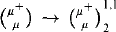Hostname: page-component-5db6c4db9b-5lzww Total loading time: 0 Render date: 2023-03-26T21:29:37.590Z Has data issue: true Feature Flags: { "useRatesEcommerce": false } hasContentIssue true

# A strong polarized relation

Published online by Cambridge University Press:  12 March 2014

## Abstract

We prove that the strong polarized relationis consistent with ZFC, for a singular μ which is a limit of measurable cardinals.

## Keywords

Type
Research Article
Information
The Journal of Symbolic Logic , September 2012 , pp. 766 - 776

## Access options

Get access to the full version of this content by using one of the access options below. (Log in options will check for institutional or personal access. Content may require purchase if you do not have access.)

## References

#### REFERENCES

 Čudnovskiĭ, G. V., Combinatorial properties of compact cardinals, Infinite and finite sets (Colloquium, Keszthely, 1973; dedicated to P. Erdős on his 60th birthday), Vol. I, North-Holland, Amsterdam, 1975, pp. 289306. Colloquium of the János Bolyai Mathematical Society. Vol. 10.Google Scholar
 Erdős, P., Hajnal, A., and Rado, R., Partition relations for cardinal numbers, Acta Mathematica Academiae Scientiarum Hungaricae, vol. 16 (1965), pp. 93196.CrossRefGoogle Scholar
 Erdős, P. and Rado, R., A partition calculus in set theory, Bulletin of the American Mathematical Society, vol. 62 (1956), pp. 427489.CrossRefGoogle Scholar
 Foreman, M. and Hajnal, A., A partition relation for successors of large cardinals, Mathematische Annalen, vol. 325 (2003), no. 3, pp. 583623.CrossRefGoogle Scholar
 Gitik, M. and Shelah, S., On densities of box products, Topology and its Applications, vol. 88 (1998), no. 3, pp. 219237.CrossRefGoogle Scholar
 Laver, R., Making the supercompactness of k indestructible under k-directed closed forcing, Israel Journal of Mathematics, vol. 29 (1978), no. 4, pp. 385388.CrossRefGoogle Scholar
 Magidor, M., Changing cofinality of cardinals, Polska Akademia Nauk. Fundamenta Mathematicae, vol. 99 (1978), no. 1, pp. 6171.Google Scholar
 Shelah, S., A weak generalization of MA to higher cardinals, Israel Journal of Mathematics, vol. 30 (1978), no. 4, pp. 297306.CrossRefGoogle Scholar
 Shelah, S., More on cardinal arithmetic, Archive for Mathematical Logic, vol. 32 (1993), no. 6, pp. 399428.CrossRefGoogle Scholar
 Shelah, S., Cardinal arithmetic, Oxford Logic Guides, vol. 29, The Clarendon Press, Oxford University Press, New York, 1994.Google Scholar
 Shelah, S., A polarized partition relation and failure of GCH at singular strong limit, Fundamenta Mathematicae, vol. 155 (1998), no. 2, pp. 153160.Google Scholar
 Shelah, S., On con(dominatingλ > covλ(meagre)), preprint.+covλ(meagre)),+preprint.>Google Scholar
 Williams, N. H., Combinatorial set theory, studies in logic and the foundations of mathematics, vol. 91, North-Holland, Amsterdam, 1977.Google Scholar